Home » GENERATESERIES Function DAX

# GENERATESERIES Function DAX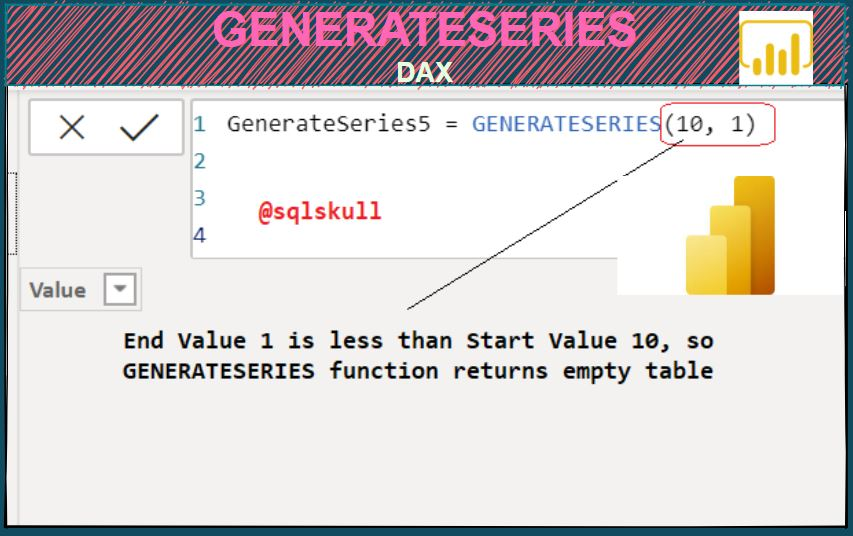### GENERATESERIES function is a Power BI Table Manipulation function in DAX which returns a table with one column which contains sequential values from start to end.

SYNTAX

`GENERATESERIES(<start_value>, <end_value>[, <increment_value>])`

start_value is the initial value used to generate the sequence.
end_value is the end value used to generate the sequence.
increment_value is an Optional. It is an increment value of the sequence. When it is not provided, the default value is 1.

Following are some key points on GENERATESERIES function as follows:

• The name of the column in table that contains the sequential values is Value.
• Each sequential values differs from the preceding value by a constant quantity.
• GENERATESERIES function returns an empty table when end value is less than start value.
• Increment value must be a positive value.

Lets look at an example using GENERATESERIES function in Power BI.

For this we will create a new table, go to Modeling tab in Ribbon bar and select New table to write a DAX expression as shown below.Lets see GENERATESERIES function in action.

#### Generating a sequence of integer values using GENERATESERIES function

Following statement generates a table which contains sequence of integer values from 1 to 20.

`GenerateSeries1 = GENERATESERIES(1, 20)`

The first arguments define the start value of sequence and second argument define the end value of sequence.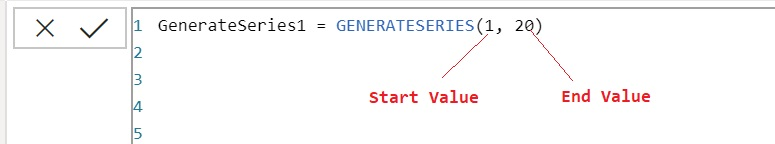Once you commit the DAX, it creates a single column table in Data model as shown below.

You can see, GENERATESERIES function create a table named GenerateSeries1, which contains single column named Value.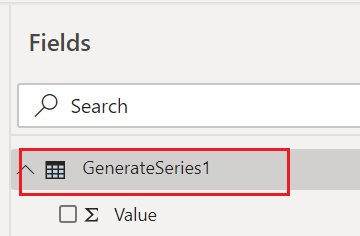Now we will verify the data in table, you can either drag the table data into visual or can check in data view.

Lets go to data view to see the data generated by GENRETAESERIES function.

You can see, it creates a single column table that contains the sequential value starting from 1 to 20.

`GenerateSeries1 = GENERATESERIES(1, 20)`#### Generating a sequence of integer values with specified increment value using GENERATESERIES function

You can also specified an incremental value for sequence. For this you need to provide third argument value in GENERATESERIES function, which defines the increment value for sequence, it must be a positive number.

Following DAX uses GENERATESERIES function, which returns a sequence of Integer values starting with 1 and incremented by 2 up to 20.

Once you commit the DAX, it returns a single column table named GenerateSeries2.

You can see the sequence value is started from 1 up to 19.

You will be wondering why the last value is not 20 ?

The reason is that sequence generated by GENERATESERIES function is stopped at the last value that may be less than or equal to given end value.

Here sequence value is incremented by 2 so the next increment value after 19 is 21 which is greater than the end value that is 20 so the sequence stops at 19.

`GenerateSeries2 = GENERATESERIES(1, 20, 2)`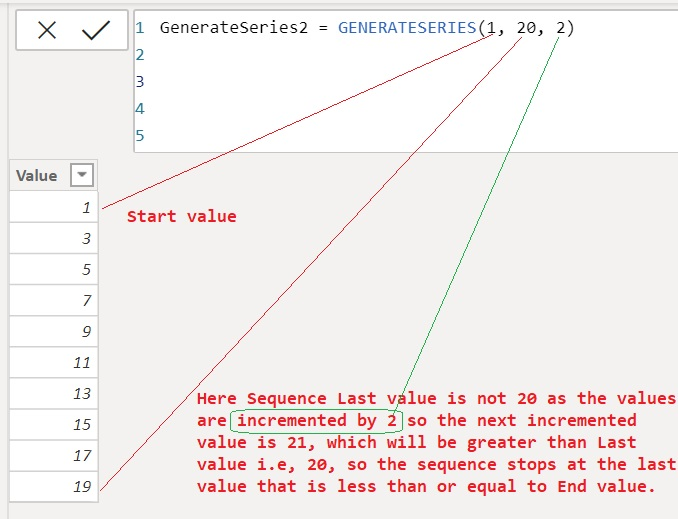#### Generating sequence starting with negative integer values to positive integer values

You can specified negative start and end value in GENERATESERIES function.

Following DAX uses GENERATESERIES function, which generates the Sequence value starting from -5 to 5.

`GenerateSeries3 = GENERATESERIES(-5, 5, 1)`#### Specifying negative increment value in GENERATESERIES function

GENERERATESERIES function gives an error for negative increment value as shown below.

`GenerateSeries4 = GENERATESERIES(1, 5, -1)`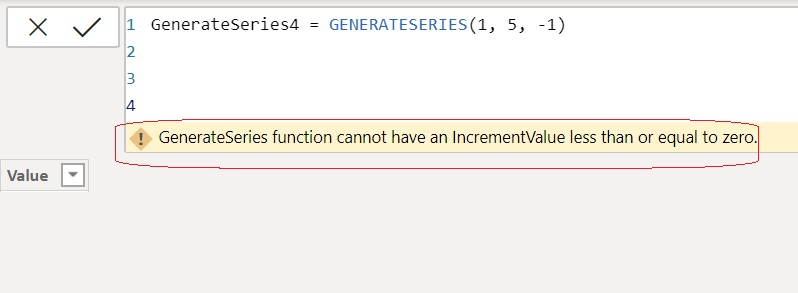#### GENERATESERIES function on specifying end value that is less than start value

GENERATESERIES function return empty table when End value is less than start value as shown below Start value is 10 while End value is 1.

`GenerateSeries5 = GENERATESERIES(10, 1)`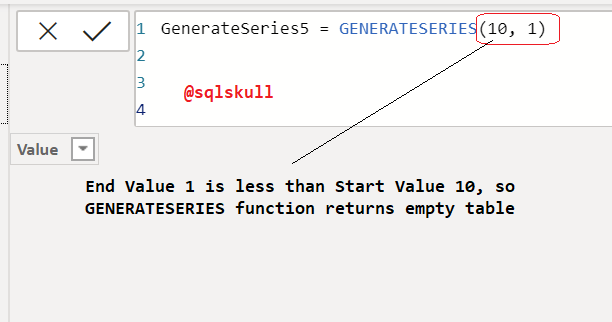SUMMARIZE

SUMMARIZECOLUMNS

VALUES

DATATABLE